6227.0 - Education and Work, Australia, May 2012
ARCHIVED ISSUE Released at 11:30 AM (CANBERRA TIME) 29/11/2012
 Page tools: .mffeedback,#pjs { display :none; } Enable Javascript to Print PagesPrint AllEmail Notification Explanatory Notes Glossary Abbreviations Quality Declaration - Summary Data quality (Technical Note) TECHNICAL NOTE DATA QUALITY RELIABILITY OF THE ESTIMATES 1 Since the estimates in this publication are based on information obtained from a sample, they are subject to sampling variability. That is, they may differ from those estimates that would have been produced if all dwellings had been included in the survey. One measure of the likely difference is given by the standard error (SE), which indicates the extent to which an estimate might have varied by chance because only a sample of dwellings (or households) was included. There are about two chances in three (67%) that a sample estimate will differ by less than one SE from the number that would have been obtained if all dwellings had been included, and about 19 chances in 20 (95%) that the difference will be less than two SEs. 2 Another measure of the likely difference is the relative standard error (RSE%), which is obtained by expressing the SE as a percentage of the estimate.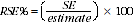3 RSE%s for the 2012 Survey of Education and Work (SEW) have been calculated using the Jackknife method of variance estimation. This involves the calculation of 30 'replicate' estimates based on 30 different sub samples of the obtained sample. The variability of estimates obtained from these subsamples is used to estimate the sample variability surrounding the estimate. 4 RSE%s of all of the estimates in this publication are included in the Data Cubes released as part of the publication and available from the Downloads tab of the publication. 5 Tables 2, 7, 8, 21 and 22 contain estimates collected from previous Education and Work surveys. The spreadsheets associated with this release contain RSE%s for these estimates. The RSE%s for the years prior to 2004 were calculated using the previous statistical SE models, which are available from each relevant issue of Education and Work, Australia (cat. no. 6227.0) available on the ABS website. For data from 2004, and later data, the RSE%s were directly calculated for each separate estimate. This method differs from that presented in the 2004 and 2005 publication, which describes using statistical SE models to calculate RSE%s for all time points. While the direct method is more accurate, the difference between the two is usually not significant for most estimates. 6 Only estimates (numbers and proportions) with RSE%s less than 25% are considered sufficiently reliable for most purposes. Estimates with RSE%s between 25% and 50% have been included and are preceded by an asterisk (e.g. *1.3) to indicate they are subject to high sample variability and should be used with caution. Estimates with RSE%s greater than 50% are preceded by a double asterisk (e.g. **0.6) to indicate that they are considered too unreliable for general use. CALCULATION OF STANDARD ERROR 7 Standard errors can be calculated using the estimates (counts or proportions) and the corresponding RSE%s. For example, Table 1 shows the estimated number of females in Victoria enrolled in study for a qualification was 374,500. The RSE Table corresponding to the estimates in Table 1 (included in the Data Cubes) shows the RSE% for this estimate is 2.4%. The SE is calculated by: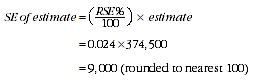8 Therefore, there are about two chances in three that the actual number of females in Victoria enrolled in a course of study was in the range of 365,500 to 383,500 and about 19 chances in 20 that the value was in the range 356,500 to 392,500. This example is illustrated in the diagram below: Calculation of Confidence Intervals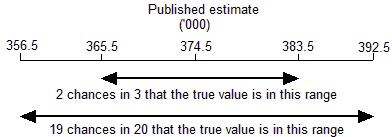PROPORTION AND PERCENTAGES 9 Proportions and percentages formed from the ratio of two estimates are also subject to sampling errors. The size of the error depends on the accuracy of both the numerator and the denominator. A formula to approximate the RSE of a proportion is given below. This formula is only valid when the numerator is a subset of the denominator.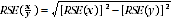10 As an example, using estimates from Table 1, of the 726,500 persons enrolled in a course of study in Victoria, 48.5%, that is, 352,000 are males. The RSE% for 726,500 is 2.1% and the RSE% for 352,000 is 2.5% (see Table 1 Relative Standard Errors). Applying the above formula, the RSE% for the percentage of males in Victoria enrolled in a course of study is: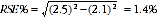11 Therefore, the SE for the percentage of males in Victoria enrolled in a course of study is 0.7 percentage points (=(1.4/100) x 48.4). Hence, there are about two chances in three that the percentage of males in Victoria enrolled in a course of study is between 47.7% and 49.1%, and 19 chances in 20 that the percentage is between 47% and 49.8%. DIFFERENCES 12 Published estimates may also be used to calculate the difference between two survey estimates (numbers or proportions). Such an estimate is also subject to sampling error. The sampling error of the difference between two estimates depends on their SEs and the relationship (correlation) between them. An approximate SE of the difference between two estimates (x-y) may be calculated by the following formula: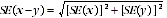13 While this formula will only be exact for differences between separate and uncorrelated characteristics or sub populations, it provides a good approximation for the differences likely to be of interest in this publication. SIGNIFICANCE TESTING 14 A statistical significance test for any comparisons between estimates can be performed to determine whether it is likely that there is a difference between two corresponding population characteristics. The standard error of the difference between two corresponding estimates (x and y) can be calculated using the formula in paragraph 11. This standard error is then used to calculate the following test statistic: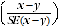15 If the value of this test statistic is greater than 1.96 then there is evidence, with a 95% level of confidence, of a statistically significant difference in the two populations with respect to that characteristic. Otherwise, it cannot be stated with confidence that there is a real difference between the populations with respect to that characteristic. OTHER SOURCES OF ERROR 16 The imprecision due to sampling variability, which is measured by the SE, should not be confused with inaccuracies that may occur because of imperfections in reporting by respondents and recording by interviewers, and errors made in coding and processing data. Inaccuracies of this kind are referred to as non-sampling error, and they occur in any enumeration, whether it be a full count or sample. Every effort is made to reduce non-sampling error to a minimum by careful design of questionnaires, intensive training and supervision of interviewers, and efficient operating procedures. Document Selection These documents will be presented in a new window.